## Friday, December 10, 2010

### Quantum superposition

Quantum mechanics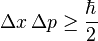$\Delta x\, \Delta p \ge \frac{\hbar}{2}$
Uncertainty principle
Introduction · Mathematical formulations
[hide]Fundamental concepts
Quantum state · Wave function
Superposition · Entanglement
Complementarity · Duality · Uncertainty
Measurement · Exclusion
Decoherence · Ehrenfest theorem · Tunnelling
......refers to the quantum mechanical property of a particle to occupy all of its possible quantum states simultaneously. Due to this property, to completely describe a particle one must include a description of every possible state and the probability of the particle being in that state.[citation needed] Since the Schrödinger equation is linear, a solution that takes into account all possible states will be a Linear combination of the solutions for each individual state.[clarification needed] This mathematical property of linear equations is known as the superposition principle.

## The Superposition principle of quantum mechanics

The principle of superposition states that if the world can be in any configuration, any possible arrangement of particles or fields, and if the world could also be in another configuration, then the world can also be in a state which is a superposition of the two, where the amount of each configuration that is in the superposition is specified by a complex number.

## Examples

For an equation describing a physical phenomenon, the superposition principle states that a linear combination of solutions to an equation is also a solution. When this is true then the equation is linear and said to obey the superposition principle. Thus if functions f1, f2, and f3 solve the linear equation ψ, then ψ=c1f1+c2f2+c3f3 would also be a solution where each c is a coefficient. For example, the electrical field due to a distribution of charged particles can be described by the vector sum of the contributions of the individual particles.

Similarly, probability theory states that the probability of an event can be described by a linear combination of the probabilities of certain specific other events (see Mathematical treatment). For example, the probability of flipping two coins (coin A and coin B) and having at least one turn face up can be expressed as the sum of the probabilities for three specific events- A heads with B tails, A heads with B heads, and A tails with B heads. In this case the probability could be expressed as:
P(heads >  = 1) = P(AnotB) + P(AandB) + P(BnotA)
or even:
P(heads >  = 1) = 1 − P(notAnotB)
Probability theory, as with quantum theory, would also require that the sum of probabilities for all possible events, not just those satisfying the previous condition, be normalized to one. Thus:
P(AnotB) + P(AandB) + P(BnotA) + P(notAnotB) = 1
Probability theory also states that the probability distribution along a continuum (i.e., the chance of finding something is a function of position along a continuous set of coordinates) or among discrete events (the example above) can be described using a probability density or unit vector respectively with the probability magnitude being given by a square of the density function.

In quantum mechanics an additional layer of analysis is introduced as the probability density function is now more specifically a wave function ψ. The wave function is either a complex function of a finite set of real variables or a complex vector formed of a finite or infinite number of components. As the coefficients in the linear combination that describes our probability density are now complex, the probability must now come from the absolute value of the multiplication of the wave function by its complex conjugate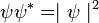$\psi \psi^* = \mid \psi \mid ^2$. In cases where the functions are not complex, the probability of an event occurring dependent on any member of a subset of the complete set of possible events occurring is the simple sum of the event probabilities in that subset. For example, if an observer rings a bell whenever one or more coins land hands up in the example above, then the probability of the observer ringing a bell is the same as the sum of the probabilities of each event in which at least one coin lands heads up. This is a simple sum since the square of the probability function describing this system is always positive. Using the wave equation, the multiplication of the function by its complex conjugate (square) is not always positive and may produce counterintuitive results.

For example, if a photon in a plus spin state has a 0.1 amplitude to be absorbed and take an atom to the second energy level, and if the photon in a minus spin state has a −0.1 amplitude to do the same thing, a photon which has an equal amplitude to be plus or minus would have zero amplitude to take the atom to the second excited state and the atom will not be excited. If the photon's spin is measured before it reaches the atom, whatever the answer, plus or minus, it will have a nonzero amplitude to excite the atom, plus or minus 0.1.

Assuming normalization, the probability density in quantum mechanics is equal to the square of the absolute value of the amplitude. The further the amplitude is from zero, the bigger the probability. Where probability distribution is represented as a continuous function the probability is the integral of the density function over the relevant values. Where the wave equation is represented as a complex vector, then probability will be extracted from the absolute value of an inner-product of the coefficient matrix and its complex conjugate. In the atom example above, the probability that the atom will be excited is 0. But the only time probability enters the picture is when an observer gets involved. If you look to see which way the atom is, the different amplitudes become probabilities for seeing different things. So if you check to see whether the atom is excited immediately after the photon with 0 amplitude reaches it, there is no chance of seeing the atom excited.

Another example: If a particle can be in position A and position B, it can also be in a state where it is an amount "3i/5" in position A and an amount "4/5" in position B. To write this, physicists usually say: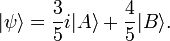$|\psi\rangle = {3\over 5} i |A\rangle + {4\over 5} |B\rangle.$
In the description, only the relative size of the different components matter, and their angle to each other on the complex plane. This is usually stated by declaring that two states which are a multiple of one another are the same as far as the description of the situation is concerned.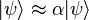$|\psi \rangle \approx \alpha |\psi \rangle$
The fundamental dynamical law of quantum mechanics is that the evolution is linear, meaning that if the state A turns into A' and B turns into B' after 10 seconds, then after 10 seconds the superposition ψ turns into a mixture of A' and B' with the same coefficients as A and B. A particle can have any position, so that there are different states which have any value of the position x. These are written: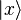$|x\rangle$
The principle of superposition guarantees that there are states which are arbitrary superpositions of all the positions with complex coefficients: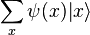$\sum_x \psi(x) |x\rangle$
This sum is defined only if the index x is discrete. If the index is over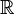$\reals$, then the sum is not defined and is replaced by an integral instead. The quantity ψ(x) is called the wavefunction of the particle.
If a particle can have some discrete orientations of the spin, say the spin can be aligned with the z axis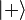$|+\rangle$ or against it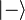$|-\rangle$, then the particle can have any state of the form: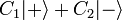$C_1 |+\rangle + C_2 |-\rangle$
If the particle has both position and spin, the state is a superposition of all possibilities for both: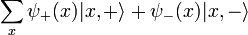$\sum_x \psi_+(x)|x,+\rangle + \psi_-(x)|x,-\rangle \,$
The configuration space of a quantum mechanical system cannot be worked out without some physical knowledge. The input is usually the allowed different classical configurations, but without the duplication of including both position and momentum.
A pair of particles can be in any combination of pairs of positions. A state where one particle is at position x and the other is at position y is written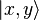$|x,y\rangle$. The most general state is a superposition of the possibilities: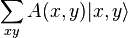$\sum_{xy} A(x,y) |x,y\rangle \,$
The description of the two particles is much larger than the description of one particle — it is a function in twice the number of dimensions. This is also true in probability, when the statistics of two random things are correlated. If two particles are uncorrelated, the probability distribution for their joint position P(x,y) is a product of the probability of finding one at one position and the other at the other position: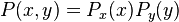$P(x,y) = P_x (x) P_y(y) \,$
In quantum mechanics, two particles can be in special states where the amplitudes of their position are uncorrelated. For quantum amplitudes, the word entanglement replaces the word correlation, but the analogy is exact. A disentangled wavefunction has the form: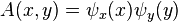$A(x,y) = \psi_x(x)\psi_y(y) \,$
while an entangled wavefunction does not have this form. Like correlation in probability, there are many more entangled states than disentangled ones. For instance, when two particles which start out with an equal amplitude to be anywhere in a box have a strong attraction and a way to dissipate energy, they can easily come together to make a bound state. The bound state still has an equal probability to be anywhere, so that each particle is equally likely to be everywhere, but the two particles will become entangled so that wherever one particle is, the other is too.

## Analogy with probability

In probability theory there is a similar principle. If a system has a probabilistic description, this description gives the probability of any configuration, and given any two different configurations, there is a state which is partly this and partly that, with positive real number coefficients, the probabilities, which say how much of each there is.

For example, if we have a probability distribution for where a particle is, it is described by the "state"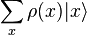$\sum_x \rho(x) |x\rangle$
Where ρ is the probability density function, a positive number that measures the probability that the particle will be found at a certain location.

The evolution equation is also linear in probability, for fundamental reasons. If the particle has some probability for going from position x to y, and from z to y, the probability of going to y starting from a state which is half-x and half-z is a half-and-half mixture of the probability of going to y from each of the options. This is the principle of linear superposition in probability.

Quantum mechanics is different, because the numbers can be positive or negative. While the complex nature of the numbers is just a doubling, if you consider the real and imaginary parts separately, the sign of the coefficients is important. In probability, two different possible outcomes always add together, so that if there are more options to get to a point z, the probability always goes up. In quantum mechanics, different possibilities can cancel.

In probability theory with a finite number of states, the probabilities can always be multiplied by a positive number to make their sum equal to one. For example, if there is a three state probability system: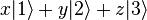$x |1\rangle + y |2\rangle + z |3\rangle \,$
where the probabilities x,y,z are positive numbers. Rescaling x,y,z so that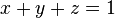$x+y+z=1 \,$
The geometry of the state space is a revealed to be a triangle. In general it is a simplex. There are special points in a triangle or simplex corresponding to the corners, and these points are those where one of the probabilities is equal to 1 and the others are zero. These are the unique locations where the position is known with certainty.

In a quantum mechanical system with three states, the quantum mechanical wavefunction is a superposition of states again, but this time twice as many quantities with no restriction on the sign: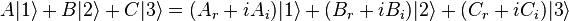$A|1\rangle + B|2\rangle + C|3\rangle = (A_r + iA_i) |1\rangle + (B_r + i B_i) |2\rangle + (C_r + iC_i) |3\rangle \,$
rescaling the variables so that the sum of the squares is 1, the geometry of the space is revealed to be a high dimensional sphere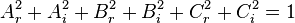$A_r^2 + A_i^2 + B_r^2 + B_i^2 + C_r^2 + C_i^2 = 1 \,$.
A sphere has a large amount of symmetry, it can be viewed in different coordinate systems or bases. So unlike a probability theory, a quantum theory has a large number of different bases in which it can be equally well described. The geometry of the phase space can be viewed as a hint that the quantity in quantum mechanics which corresponds to the probability is the absolute square of the coefficient of the superposition.

## Hamiltonian evolution

The numbers that describe the amplitudes for different possibilities define the kinematics, the space of different states. The dynamics describes how these numbers change with time. For a particle that can be in any one of infinitely many discrete positions, a particle on a lattice, the superposition principle tells you how to make a state: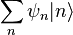$\sum_n \psi_n |n\rangle \,$
So that the infinite list of amplitudes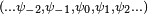$(... \psi_{-2},\psi_{-1},\psi_0,\psi_1,\psi_2 ...)$ completely describes the quantum state of the particle. This list is called the state vector, and formally it is an element of a Hilbert space, an infinite dimensional complex vector space. It is usual to represent the state so that the sum of the absolute squares of the amplitudes add up to one: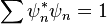$\sum \psi_n^*\psi_n = 1$
For a particle described by probability theory random walking on a line, the analogous thing is the list of probabilities (...P − 2,P − 1,P0,P1,P2,...), which give the probability of any position. The quantities that describe how they change in time are the transition probabilities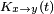$K_{x\rightarrow y}(t)$, which gives the probability that, starting at x, the particle ends up at y after time t. The total probability of ending up at y is given by the sum over all the possibilities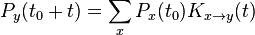$P_y(t_0+t) = \sum_x P_x(t_0) K_{x\rightarrow y}(t) \,$
The condition of conservation of probability states that starting at any x, the total probability to end up somewhere must add up to 1: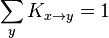$\sum_y K_{x\rightarrow y} = 1 \,$
So that the total probability will be preserved, K is what is called a stochastic matrix.
When no time passes, nothing changes: for zero elapsed time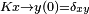$K{x\rightarrow y}(0) = \delta_{xy}$, the K matrix is zero except from a state to itself. So in the case that the time is short, it is better to talk about the rate of change of the probability instead of the absolute change in the probability.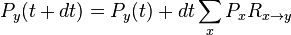$P_y(t+dt) = P_y(t) + dt \sum_x P_x R_{x\rightarrow y} \,$
where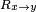$R_{x\rightarrow y}$ is the time derivative of the K matrix: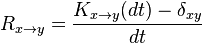$R_{x\rightarrow y} = { K_{x\rightarrow y}(dt) - \delta_{xy} \over dt} \,$.
The equation for the probabilities is a differential equation which is sometimes called the master equation: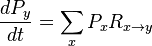${dP_y \over dt} = \sum_x P_x R_{x\rightarrow y} \,$
The R matrix is the probability per unit time for the particle to make a transition from x to y. The condition that the K matrix elements add up to one becomes the condition that the R matrix elements add up to zero: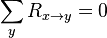$\sum_y R_{x\rightarrow y} = 0 \,$
One simple case to study is when the R matrix has an equal probability to go one unit to the left or to the right, describing a particle which has a constant rate of random walking. In this case$R_{x\rightarrow y}$ is zero unless y is either x+1,x, or x−1, when y is x+1 or x−1, the R matrix has value c, and in order for the sum of the R matrix coefficients to equal zero, the value of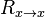$R_{x\rightarrow x}$ must be −2c. So the probabilities obey the discretized diffusion equation: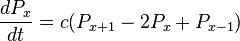${dP_x \over dt } = c(P_{x+1} - 2P_{x} + P_{x-1}) \,$
which, when c is scaled appropriately and the P distribution is smooth enough to think of the system in a continuum limit becomes: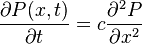${\partial P(x,t) \over \partial t} = c {\partial^2 P \over \partial x^2 } \,$
Which is the diffusion equation.
Quantum amplitudes give the rate at which amplitudes change in time, and they are mathematically exactly the same except that they are complex numbers. The analog of the finite time K matrix is called the U matrix: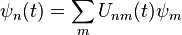$\psi_n(t) = \sum_m U_{nm}(t) \psi_m \,$
Since the sum of the absolute squares of the amplitudes must be constant, U must be unitary: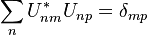$\sum_n U^*_{nm} U_{np} = \delta_{mp} \,$
or, in matrix notation,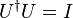$U^\dagger U = I \,$
The rate of change of U is called the Hamiltonian H, up to a traditional factor of i: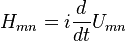$H_{mn} = i{d \over dt} U_{mn}$
The Hamiltonian gives the rate at which the particle has an amplitude to go from m to n. The reason it is multiplied by i is that the condition that U is unitary translates to the condition: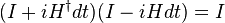$(I + i H^\dagger dt )(I - i H dt ) = I \,$$H^\dagger - H = 0 \,$
which says that H is Hermitian. The eigenvalues of the Hermitian matrix H are real quantities which have a physical interpretation as energy levels. If the factor i were absent, the H matrix would be antihermitian and would have purely imaginary eigenvalues, which is not the traditional way quantum mechanics represents observable quantities like the energy.
For a particle which has equal amplitude to move left and right, the Hermitian matrix H is zero except for nearest neighbors, where it has the value c. If the coefficient is everywhere constant, the condition that H is Hermitian demands that the amplitude to move to the left is the complex conjugate of the amplitude to move to the right. The equation of motion for ψ is the time differential equation: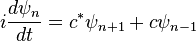$i{d \psi_n \over dt} = c^* \psi_{n+1} + c \psi_{n-1}$
In the case that left and right are symmetric, c is real. By redefining the phase of the wavefunction in time,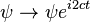$\psi\rightarrow \psi e^{i2ct}$, the amplitudes for being at different locations are only rescaled, so that the physical situation is unchanged. But this phase rotation introduces a linear term.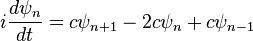$i{d \psi_n \over dt} = c \psi_{n+1} - 2c\psi_n + c\psi_{n-1}$
which is the right choice of phase to take the continuum limit. When c is very large and psi is slowly varying so that the lattice can be thought of as a line, this becomes the free Schrödinger equation: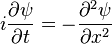$i{ \partial \psi \over \partial t } = - {\partial^2 \psi \over \partial x^2}$
If there is an additional term in the H matrix which is an extra phase rotation which varies from point to point, the continuum limit is the Schrödinger equation with a potential energy: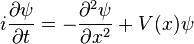$i{ \partial \psi \over \partial t} = - {\partial^2 \psi \over \partial x^2} + V(x) \psi$
These equations describe the motion of a single particle in non-relativistic quantum mechanics.

## Quantum mechanics in imaginary time

The analogy between quantum mechanics and probability is very strong, so that there are many mathematical links between them. In a statistical system in discrete time, t=1,2,3, described by a transition matrix for one time step$K_{m\rightarrow n}$, the probability to go between two points after a finite number of time steps can be represented as a sum over all paths of the probability of taking each path: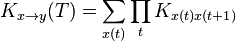$K_{x\rightarrow y}(T) = \sum_{x(t)} \prod_t K_{x(t)x(t+1)} \,$
where the sum extends over all paths x(t) with the property that x(0) = 0 and x(T) = y. The analogous expression in quantum mechanics is the path integral.

A generic transition matrix in probability has a stationary distribution, which is the eventual probability to be found at any point no matter what the starting point. If there is a nonzero probability for any two paths to reach the same point at the same time, this stationary distribution does not depend on the initial conditions. In probability theory, the probability m for the stochastic matrix obeys detailed balance when the stationary distribution ρn has the property: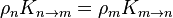$\rho_n K_{n\rightarrow m} = \rho_m K_{m\rightarrow n} \,$
Detailed balance says that the total probability of going from m to n in the stationary distribution, which is the probability of starting at m ρm times the probability of hopping from m to n, is equal to the probability of going from n to m, so that the total back-and-forth flow of probability in equilibrium is zero along any hop. The condition is automatically satisfied when n=m, so it has the same form when written as a condition for the transition-probability R matrix.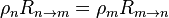$\rho_n R_{n\rightarrow m} = \rho_m R_{m\rightarrow n} \,$
When the R matrix obeys detailed balance, the scale of the probabilities can be redefined using the stationary distribution so that they no longer sum to 1: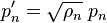$p'_n = \sqrt{\rho_n}\;p_n \,$
In the new coordinates, the R matrix is rescaled as follows: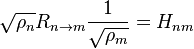$\sqrt{\rho_n} R_{n\rightarrow m} {1\over \sqrt{\rho_m}} = H_{nm} \,$
and H is symmetric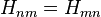$H_{nm} = H_{mn} \,$
This matrix H defines a quantum mechanical system: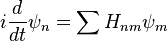$i{d \over dt} \psi_n = \sum H_{nm} \psi_m \,$
whose Hamiltonian has the same eigenvalues as those of the R matrix of the statistical system. The eigenvectors are the same too, except expressed in the rescaled basis. The stationary distribution of the statistical system is the ground state of the Hamiltonian and it has energy exactly zero, while all the other energies are positive. If H is exponentiated to find the U matrix: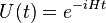$U(t) = e^{-iHt} \,$
and t is allowed to take on complex values, the K' matrix is found by taking time imaginary.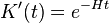$K'(t) = e^{-Ht} \,$
For quantum systems which are invariant under time reversal the Hamiltonian can be made real and symmetric, so that the action of time-reversal on the wave-function is just complex conjugation. If such a Hamiltonian has a unique lowest energy state with a positive real wave-function, as it often does for physical reasons, it is connected to a stochastic system in imaginary time. This relationship between stochastic systems and quantum systems sheds much light on supersymmetry.

## Formal interpretation

Applying the superposition principle to a quantum mechanical particle, the configurations of the particle are all positions, so the superpositions make a complex wave in space. The coefficients of the linear superposition are a wave which describes the particle as best as is possible, and whose amplitude interferes according to the Huygens principle.

For any physical quantity in quantum mechanics, there is a list of all the states where the quantity has some value. These states are necessarily perpendicular to each other using the Euclidean notion of perpendicularity which comes from sums-of-squares length, except that they also must not be i multiples of each other. This list of perpendicular states has an associated value which is the value of the physical quantity. The superposition principle guarantees that any state can be written as a combination of states of this form with complex coefficients.
Write each state with the value q of the physical quantity as a vector in some basis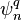$\psi^q_n$, a list of numbers at each value of n for the vector which has value q for the physical quantity. Now form the outer product of the vectors by multiplying all the vector components and add them with coefficients to make the matrix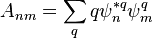$A_{nm} = \sum_q q \psi^{*q}_n \psi^q_m$
where the sum extends over all possible values of q. This matrix is necessarily symmetric because it is formed from the orthogonal states, and has eigenvalues q. The matrix A is called the observable associated to the physical quantity. It has the property that the eigenvalues and eigenvectors determine the physical quantity and the states which have definite values for this quantity.

Every physical quantity has a Hermitian linear operator associated to it, and the states where the value of this physical quantity is definite are the eigenstates of this linear operator. The linear combination of two or more eigenstates results in quantum superposition of two or more values of the quantity. If the quantity is measured, the value of the physical quantity will be random, with a probability equal to the square of the coefficient of the superposition in the linear combination. Immediately after the measurement, the state will be given by the eigenvector corresponding to the measured eigenvalue.

It is natural to ask why "real" (macroscopic, Newtonian) objects and events do not seem to display quantum mechanical features such as superposition. In 1935, Erwin Schrödinger devised a well-known thought experiment, now known as Schrödinger's cat, which highlighted the dissonance between quantum mechanics and Newtonian physics, where only one configuration occurs, although a configuration for a particle in Newtonian physics specifies both position and momentum.
In fact, quantum superposition results in many directly observable effects, such as interference peaks from an electron wave in a double-slit experiment. The superpositions, however, persist at all scales, absent a mechanism for removing them. This mechanism can be philosophical as in the Copenhagen interpretation, or physical.

Recent research indicates that chlorophyll within plants appears to exploit the feature of quantum superposition to achieve greater efficiency in transporting energy, allowing pigment proteins to be spaced further apart than would otherwise be possible.

If the operators corresponding to two observables do not commute, they have no simultaneous eigenstates and they obey an uncertainty principle. A state where one observable has a definite value corresponds to a superposition of many states for the other observable.